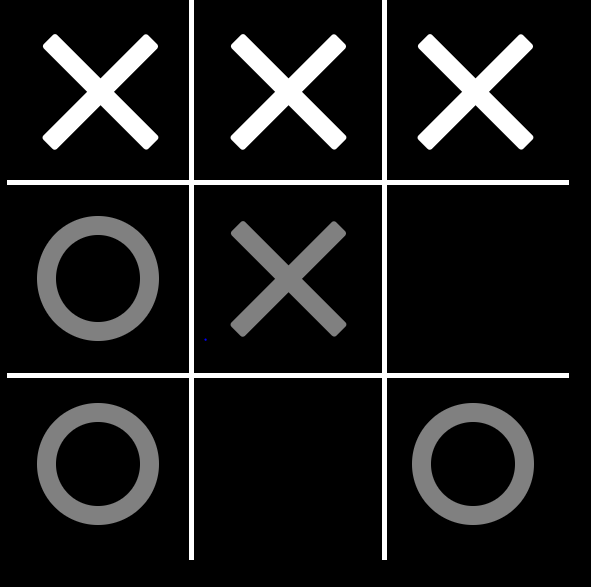# Can you beat this bot?

Original article was published by Pratyush Khare on Artificial Intelligence on Medium# Introduction to Minimax Algorithm:

Minimax is a kind of backtracking algorithm that is used in decision making and game theory to find the optimal move for a player, assuming that your opponent also plays optimally. It is widely used in two player turn-based games such as Tic-Tac-Toe, Backgammon, Mancala, Chess, etc.

For two player games, the minimax algorithm is such a tactic, which uses the fact that the two players are working towards opposite goals to make predictions about which future states will be reached as the game progresses, and then proceeds accordingly to optimize its chance of victory. The theory behind minimax is that the algorithm’s opponent will be trying to minimize whatever value the algorithm is trying to maximize (hence, “minimax”). Thus, the computer should make the move which leaves its opponent capable of doing the least damage.

• Mini-Max algorithm uses recursion to search through the game-tree.
• In this algorithm two players play the game, one is called MAX and other is called MIN.
• Both the players fight it as the opponent player gets the minimum benefit while they get the maximum benefit.
• Both Players of the game are opponent of each other, where MAX will select the maximized value and MIN will select the minimized value.
• The minimax algorithm performs a depth-first search algorithm for the exploration of the complete game tree.
• The minimax algorithm proceeds all the way down to the terminal node of the tree, then backtrack the tree as the recursion.

The general process of the Minimax algorithm is as follows:

Step 1: First, generate the entire game tree starting with the current position of the game all the way upto the terminal states. This is how the game tree looks like for the game tic-tac-toe.

Step 2: The reward of the action to reach terminal node gives the utility values for all the terminal states.
Step 3: Determine the rewards of the higher nodes with the help of the rewards of the terminal nodes. For instance, in the diagram below, we have the rewards for the terminal states written in the squares.

Let us calculate the reward for the left node(red) of the layer above the terminal. Since it is the move of the player MIN, we will choose the minimum of all the utilities. For this case, we have to evaluate MIN{3, 5, 10}, which we know is certainly 3. So the reward for the red node is 3.

Similarly, for the green node in the same layer, we will have to evaluate MIN{2,2} which is 2.

Step 4: Calculate the reward values with the help of leaves considering one layer at a time until the root of the tree.
Step 5: Eventually, all the backed-up values reach to the root of the tree, i.e., the topmost point. At that point, MAX has to choose the highest value.

In our example, we only have 3 layers so we immediately reached to the root but in actual games, there will be many more layers and nodes. So we have to evaluate MAX{3,2} which is 3.

Therefore, the best opening move for MAX is the left node(or the red one). This move is called the minimax decision as it maximizes the reward following the assumption that the opponent is also playing optimally to minimize it.

To summarize,

Minimax Decision = MAX{MIN{3,5,10},MIN{2,2}}
= MAX{3,2}
= 3